# Translate from other heatmap functions to ComplexHeatmap

In my previous post “Translate from pheatmap to ComplexHeatmap”, I introduced a ComplexHeatmap::pheatmap() which smoothly executes pheatmap::pheatmap() code without any problem. With the newly developed InteractiveComplexHeatmap package, the output of pheatmap() can be directly exported as an interactive Shiny app.

library(ComplexHeatmap)
ht = pheatmap(...)
library(InteractiveComplexHeatmap)
ht_shiny(ht)

To facilitate the users who are still using heatmap() and heatmap.2() functions, to make the output of these two functions can be exported as interactive Shiny apps as well, I implemented two similar translation functions ComplexHeatmap::heatmap() and ComplexHeatmap::heatmap.2() which use the same set of arguments as the original functions and generate almost identical plots.

Some arguments in the two original functions are ignored in the ComplexHeatmap implementation, but there won’t be any error thrown out.

Then with ComplexHeatmap::heatmap() and ComplexHeatmap::heatmap.2(), you can make your old heatmaps interactive by:

library(ComplexHeatmap)
ht = heatmap(...) # or ht = heatmap.2(...)
library(InteractiveComplexHeatmap)
ht_shiny(ht)

To replace the two original functions with ComplexHeatmap::heatmap() and ComplexHeatmap::heatmap.2(), we need to make sure the new homonymous functions generates the same plots as the old ones. In the following examples, compare_heatmap() basically sends all the arguments to stats::heatmap() and ComplexHeatmap::heatmap() separately and you can directly compare the two plots generated by the two functions.

library(ComplexHeatmap)

## Translate from stats::heatmap()

x  = as.matrix(mtcars)
rc = rainbow(nrow(x), start = 0, end = 0.3)
cc = rainbow(ncol(x), start = 0, end = 0.3)
compare_heatmap(x, col = cm.colors(256), scale = "column",
RowSideColors = rc, ColSideColors = cc, margins = c(5,10),
xlab = "specification variables", ylab =  "Car Models",
main = "heatmap(<Mtcars data>, ..., scale = \"column\")")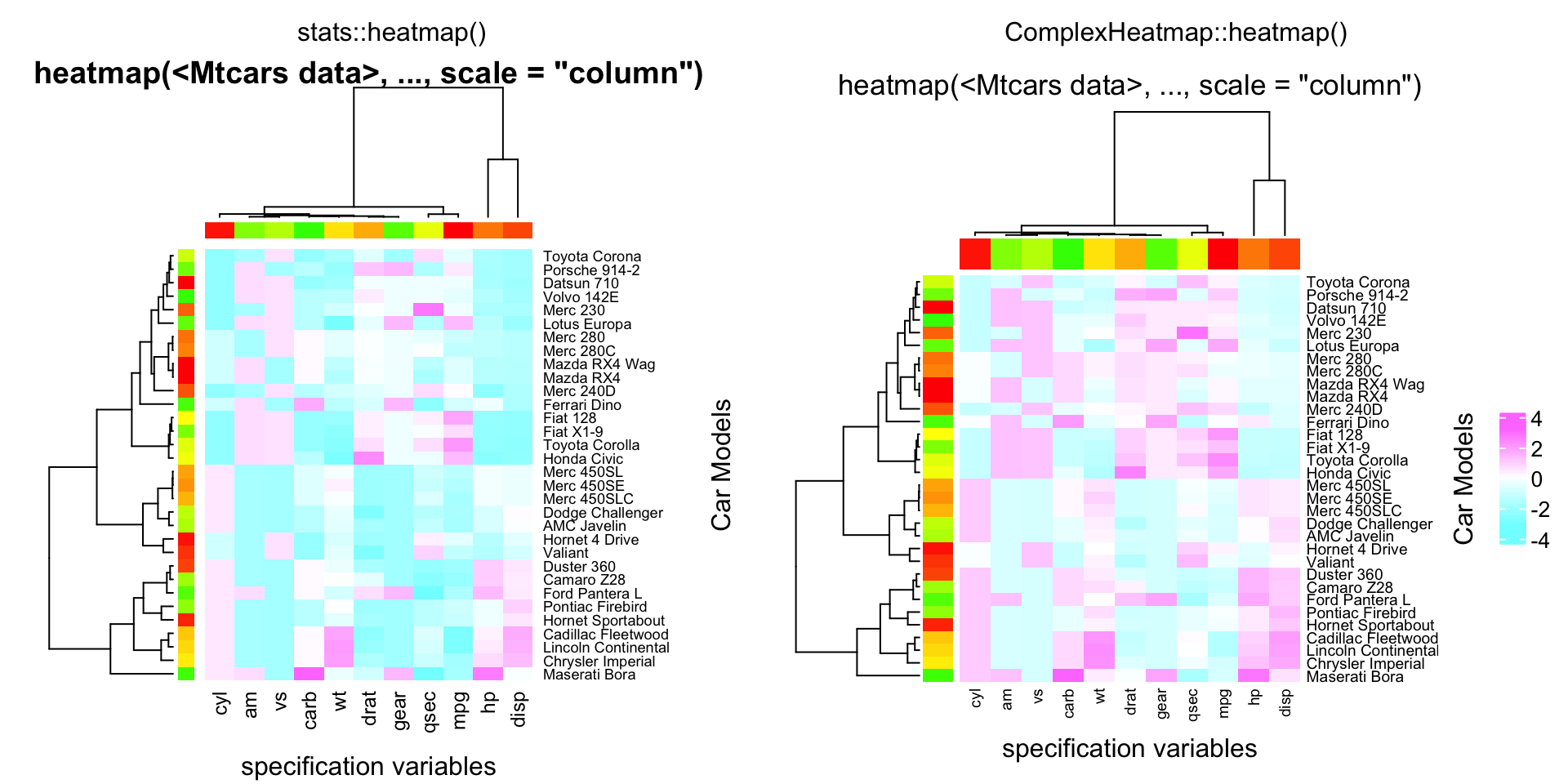compare_heatmap(x, Colv = NA, col = cm.colors(256), scale = "column",
RowSideColors = rc, margins = c(5,10),
xlab = "specification variables", ylab =  "Car Models",
main = "heatmap(<Mtcars data>, ..., scale = \"column\")")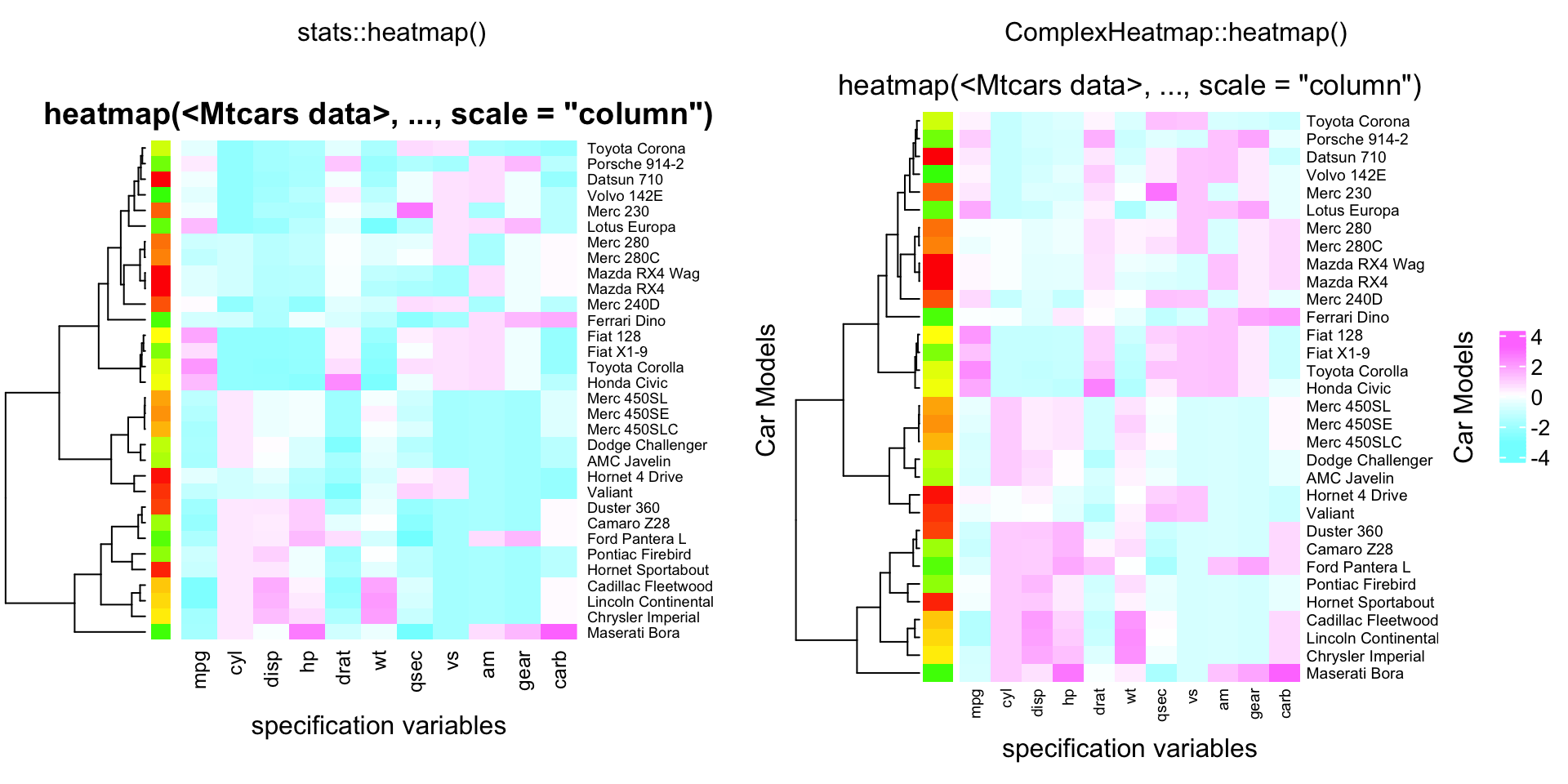compare_heatmap(x, Rowv = NA, Colv = NA, scale = "column",
main = "heatmap(*, NA, NA) ~= image(t(x))")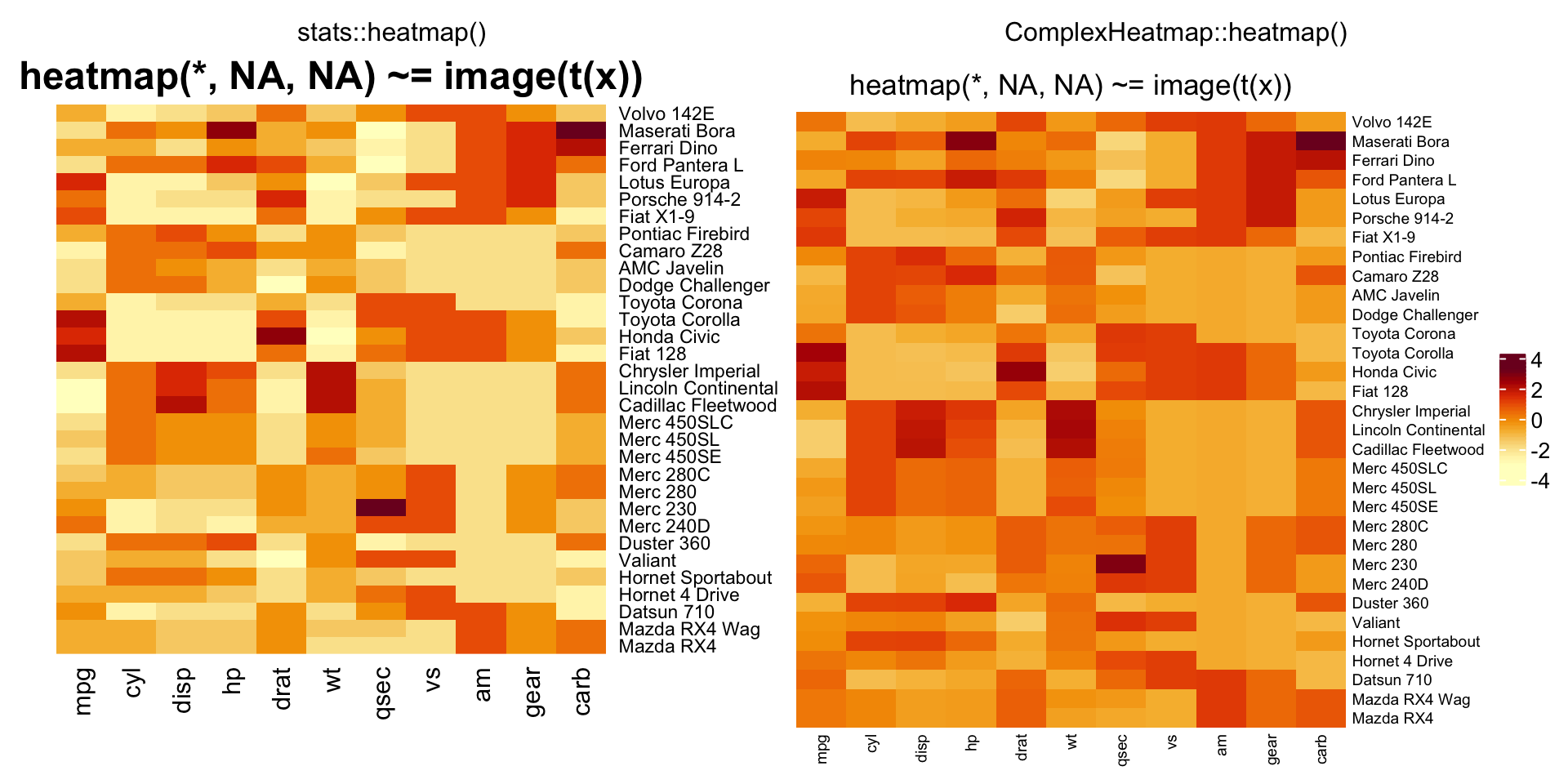Ca = cor(attitude)
cc = rainbow(nrow(Ca))
compare_heatmap(Ca, Rowv = FALSE, RowSideColors = cc, ColSideColors = cc)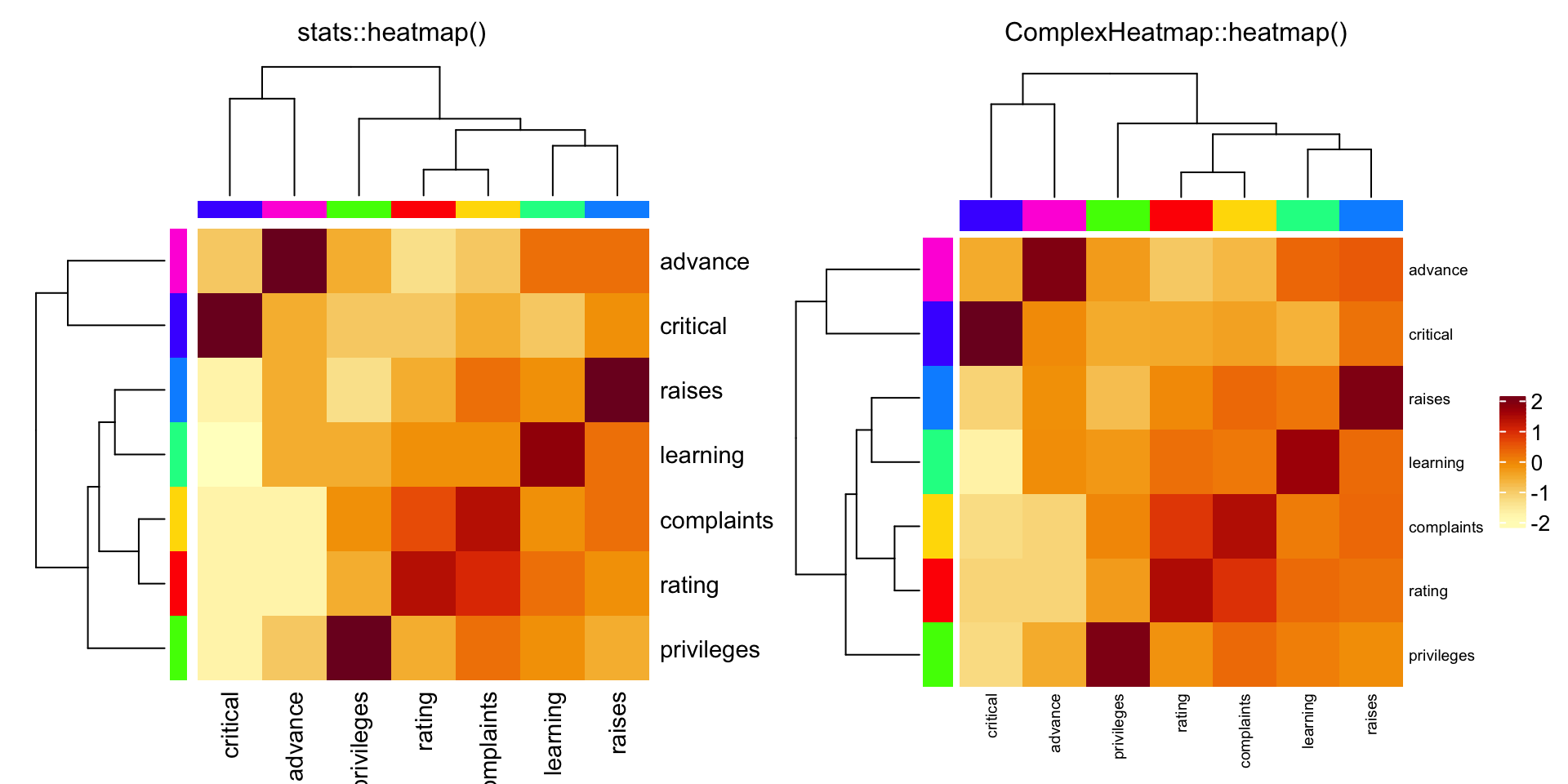cU = cor(USJudgeRatings)
compare_heatmap(cU, Rowv = FALSE, col = topo.colors(16),
distfun = function(c) as.dist(1 - c), keep.dendro = TRUE)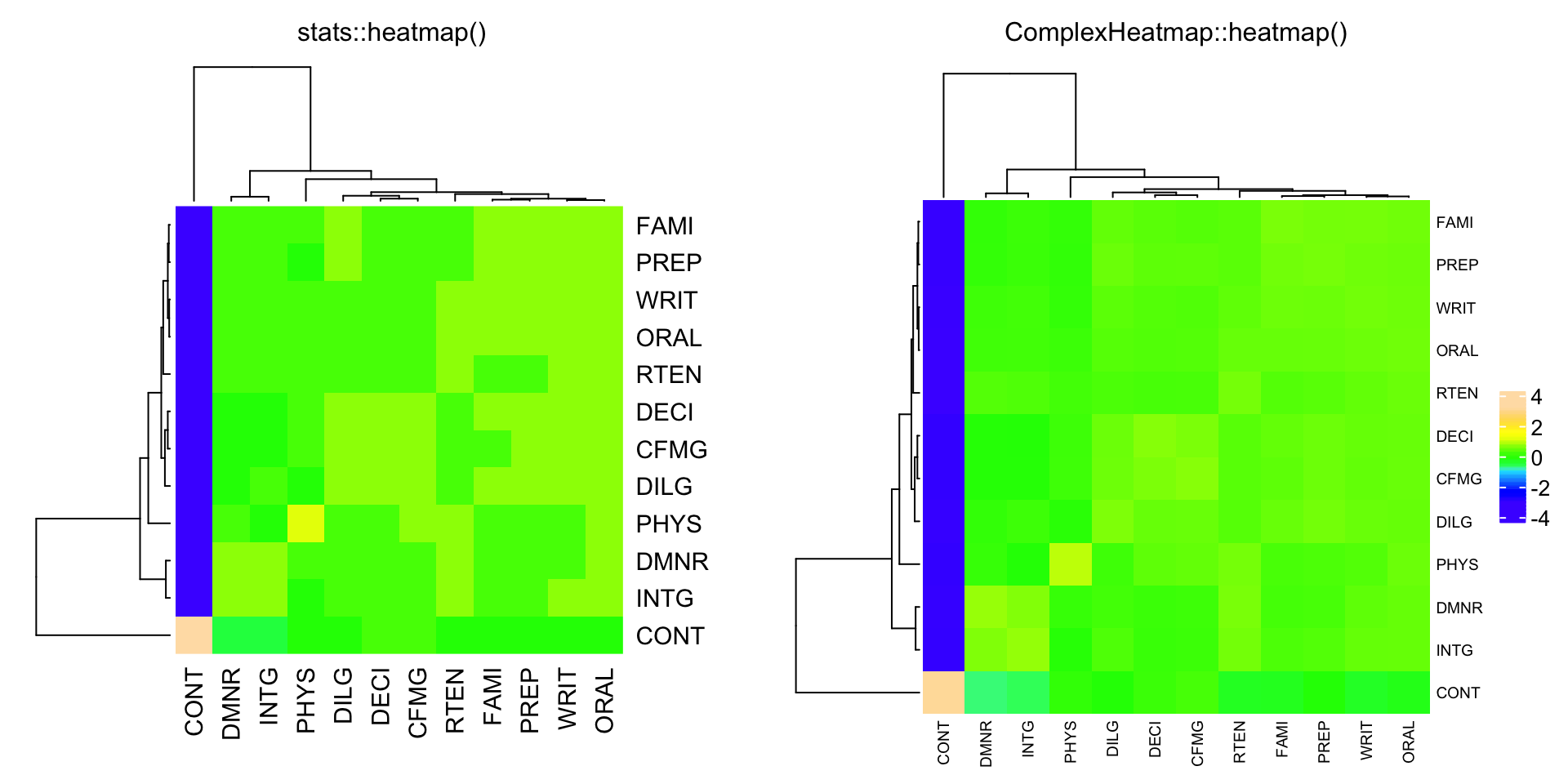## Translate from gplots::heatmap.2()

Similarly, in the following examples, compare_heatmap.2() basically sends all the arguments to gplots::heatmap.2() and ComplexHeatmap::heatmap.2() separately.

data(mtcars)
x = as.matrix(mtcars)
rc = rainbow(nrow(x), start = 0, end = .3)
cc = rainbow(ncol(x), start = 0, end = .3)
compare_heatmap.2(x)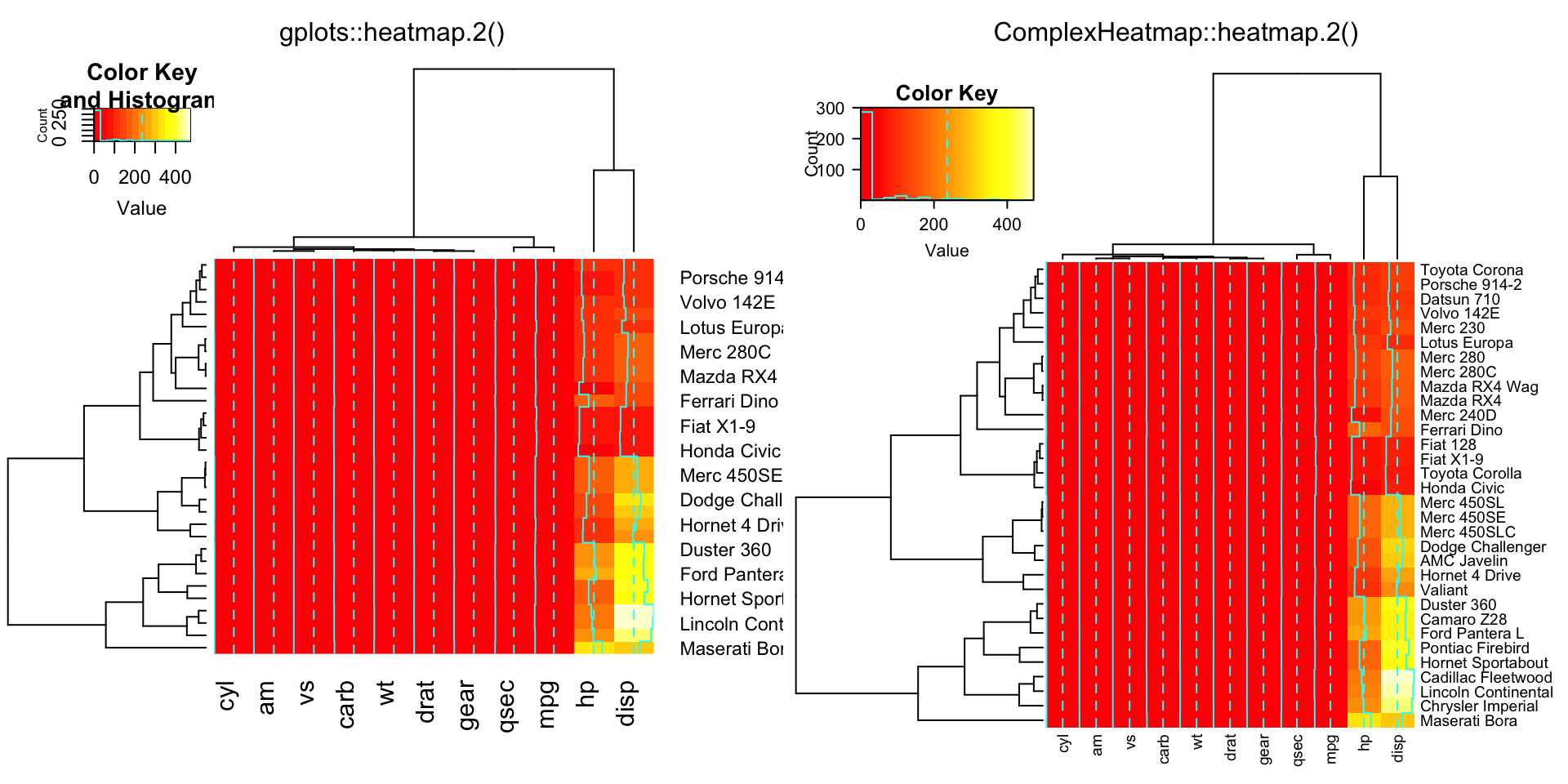compare_heatmap.2(x, dendrogram = "none")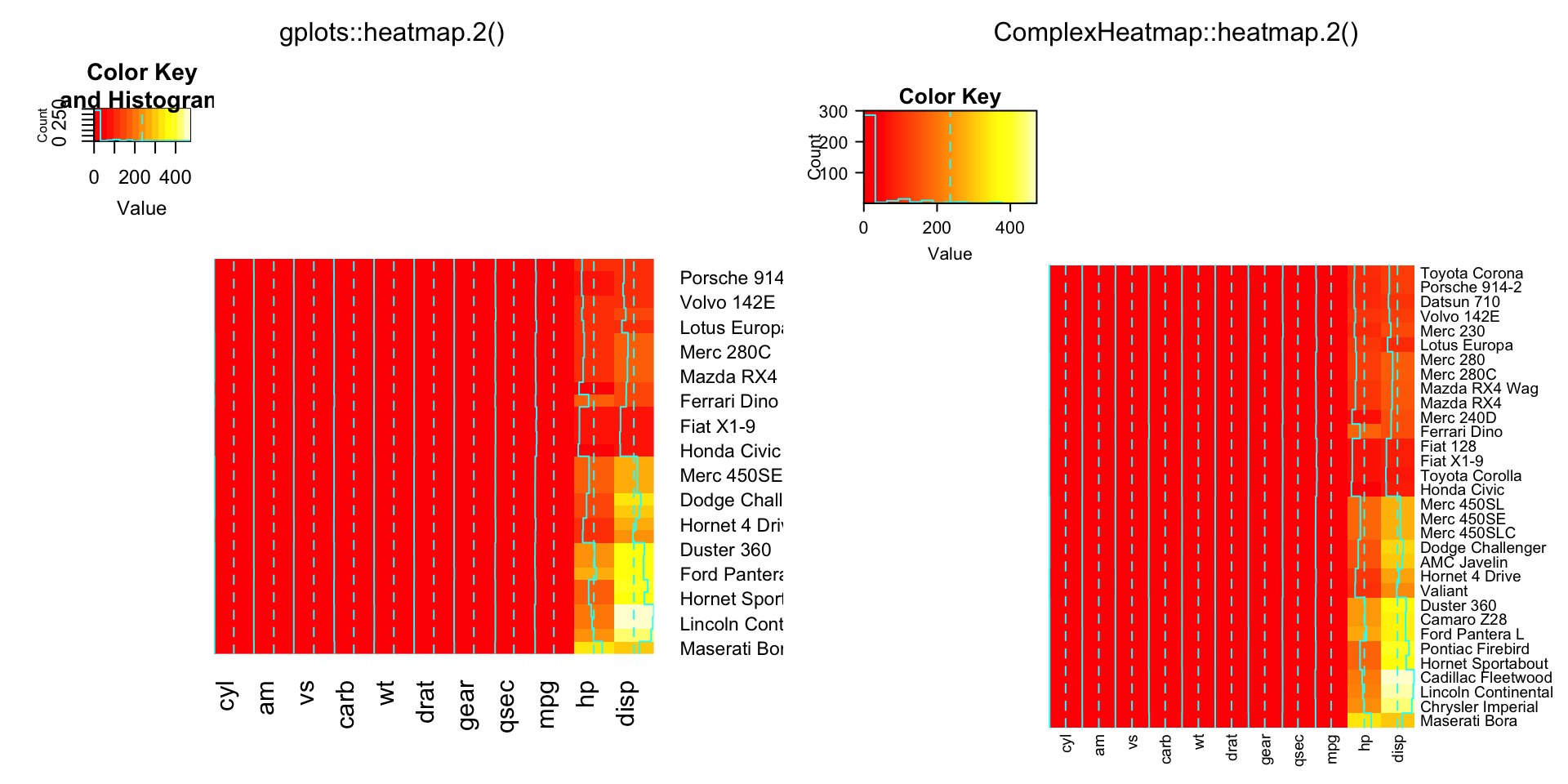compare_heatmap.2(x, dendrogram = "row")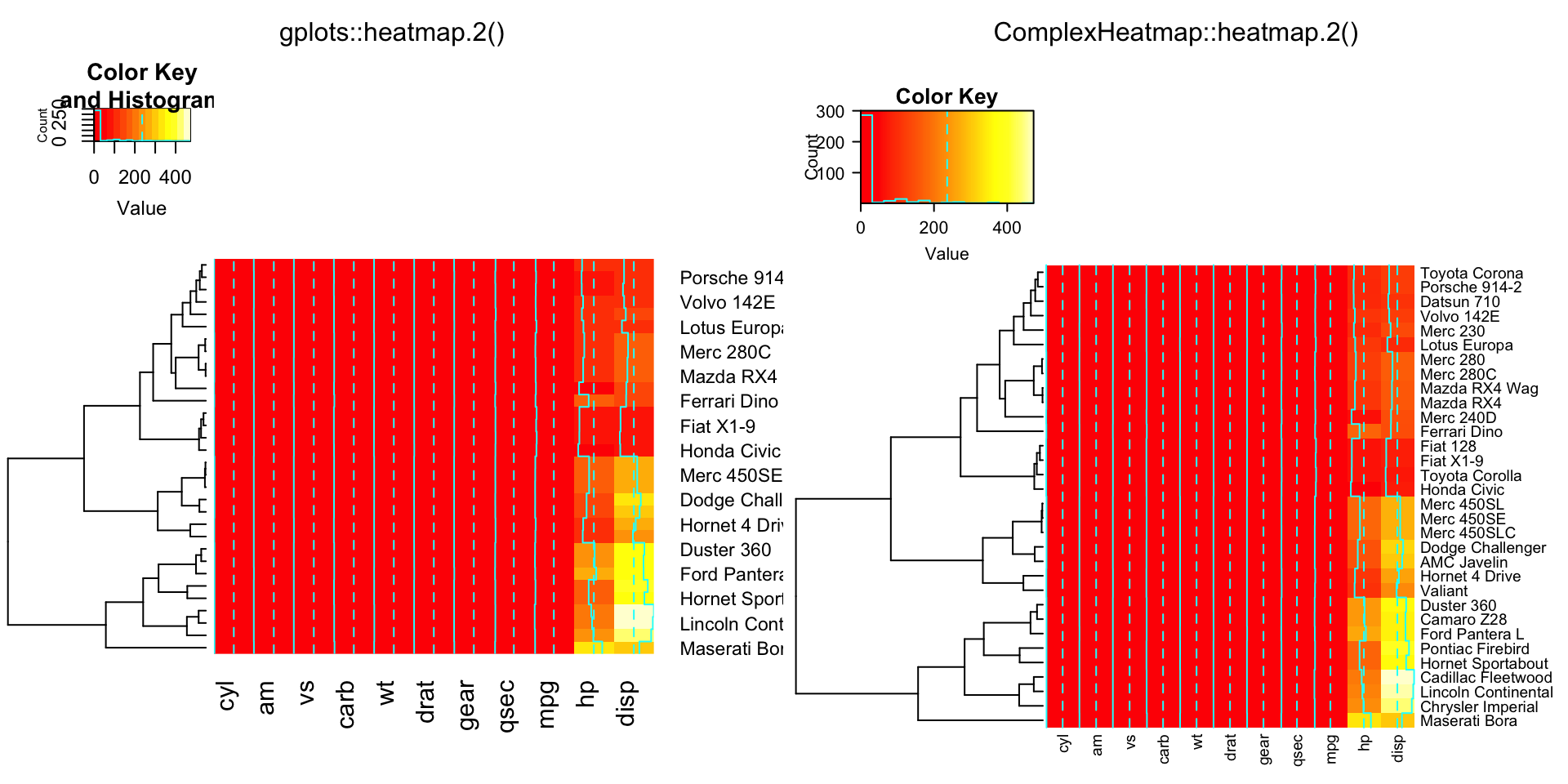compare_heatmap.2(x, dendrogram = "col")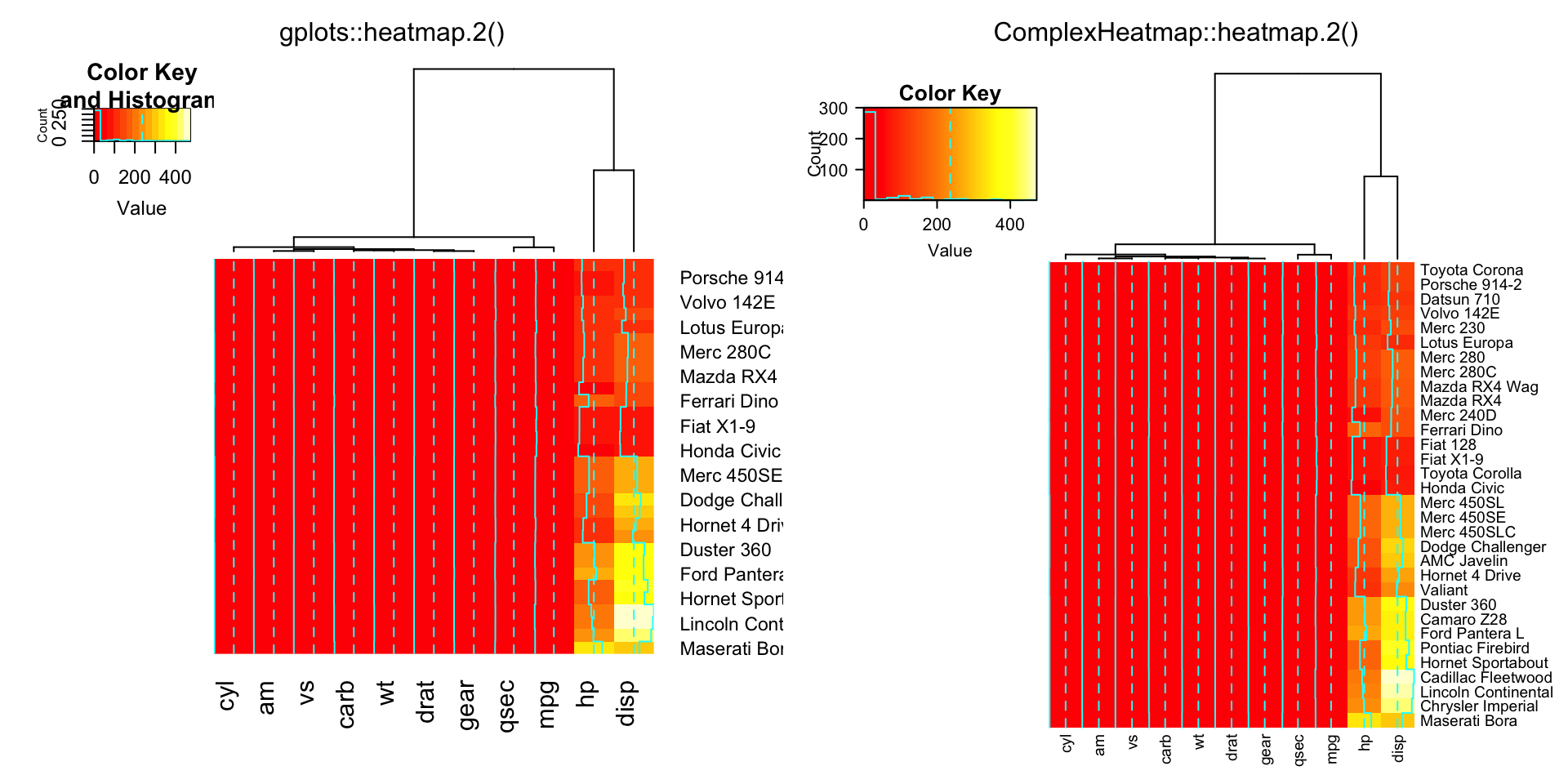compare_heatmap.2(x, Rowv = FALSE, dendrogram = "both")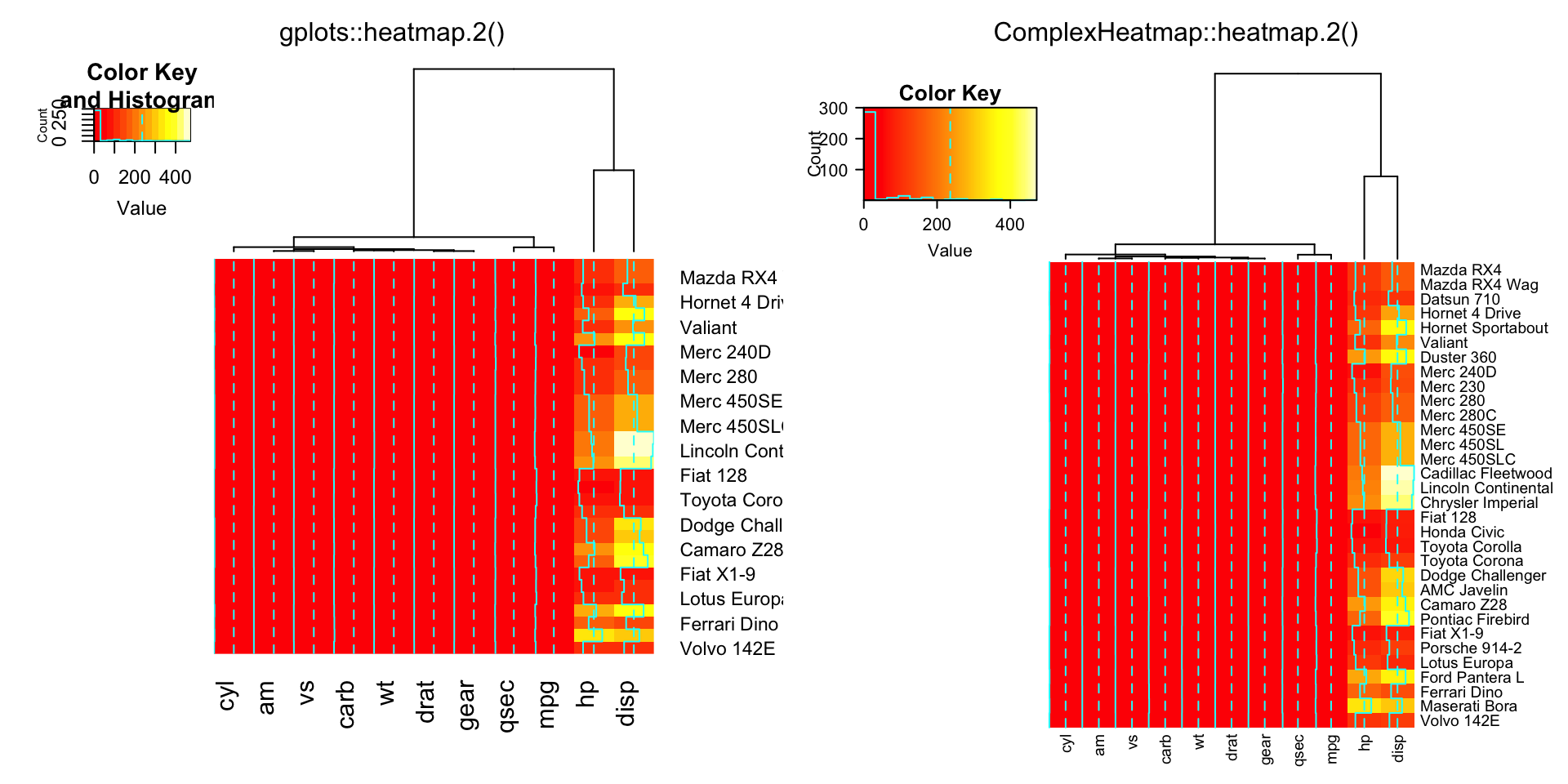compare_heatmap.2(x, Rowv = NULL, dendrogram = "both")compare_heatmap.2(x, Colv = FALSE, dendrogram = "both")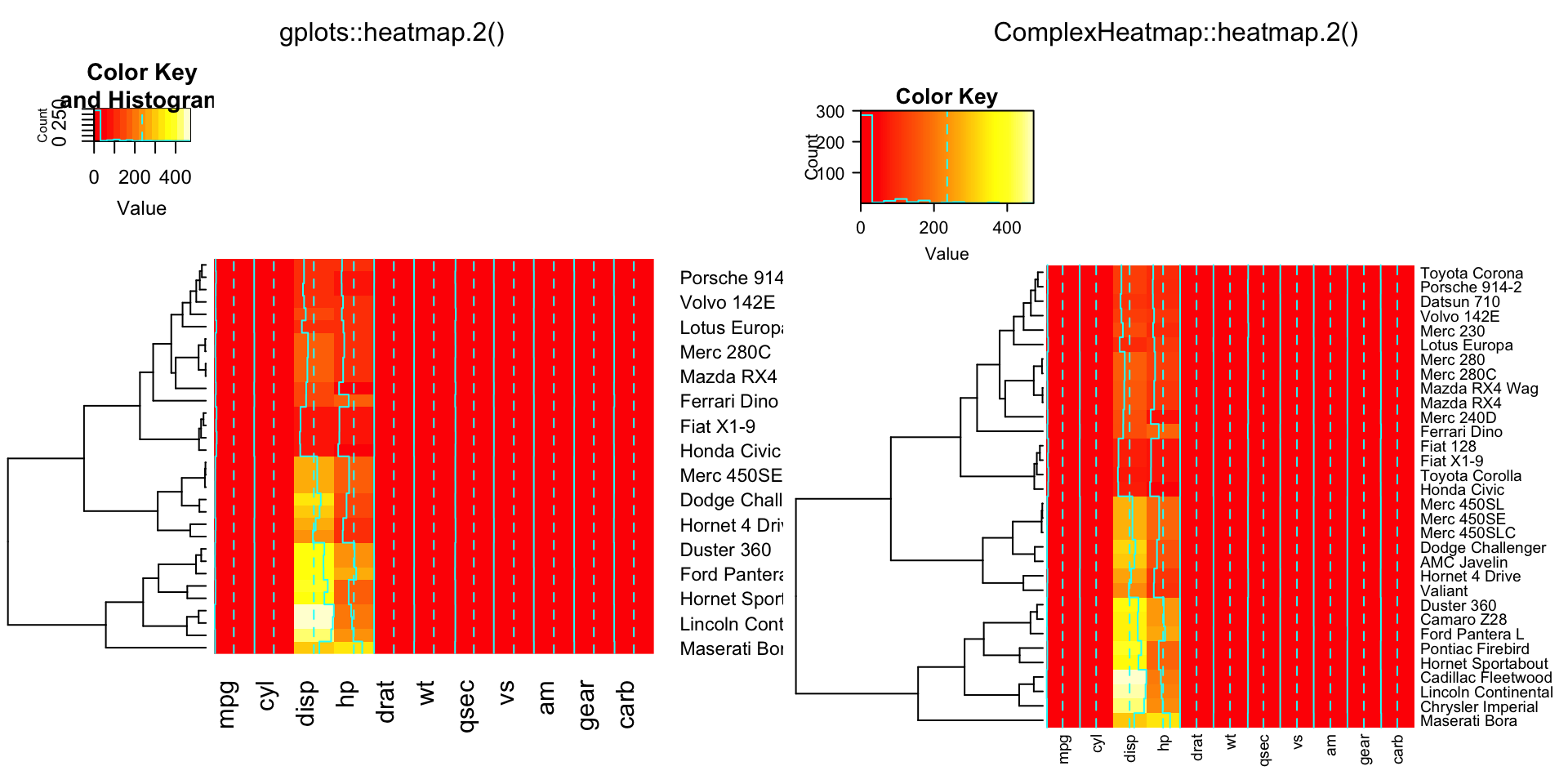compare_heatmap.2(x, reorderfun = function(d, w) reorder(d, w, agglo.FUN = mean))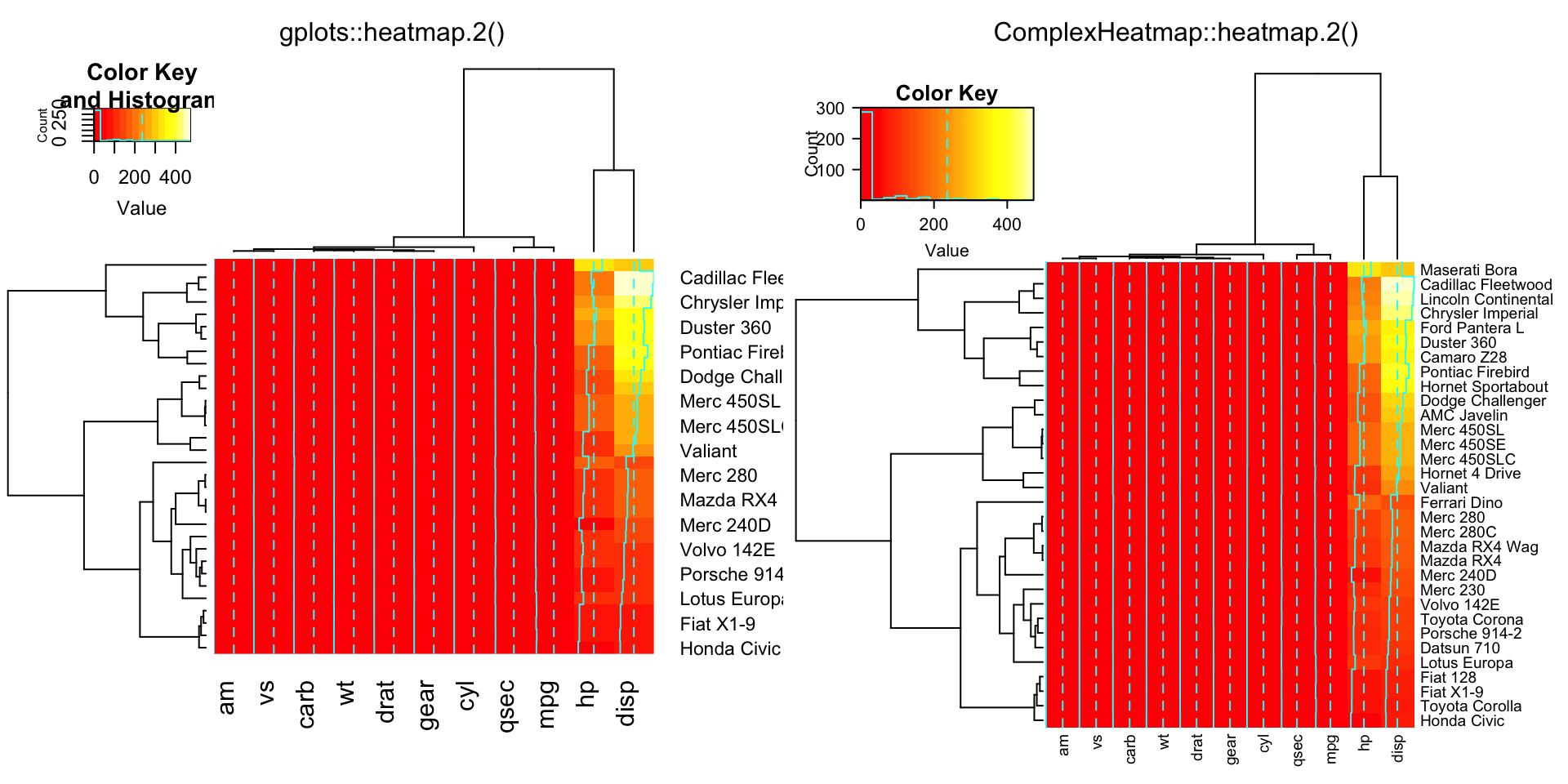compare_heatmap.2(x, col = gplots::bluered, scale = "column", tracecol = "#303030")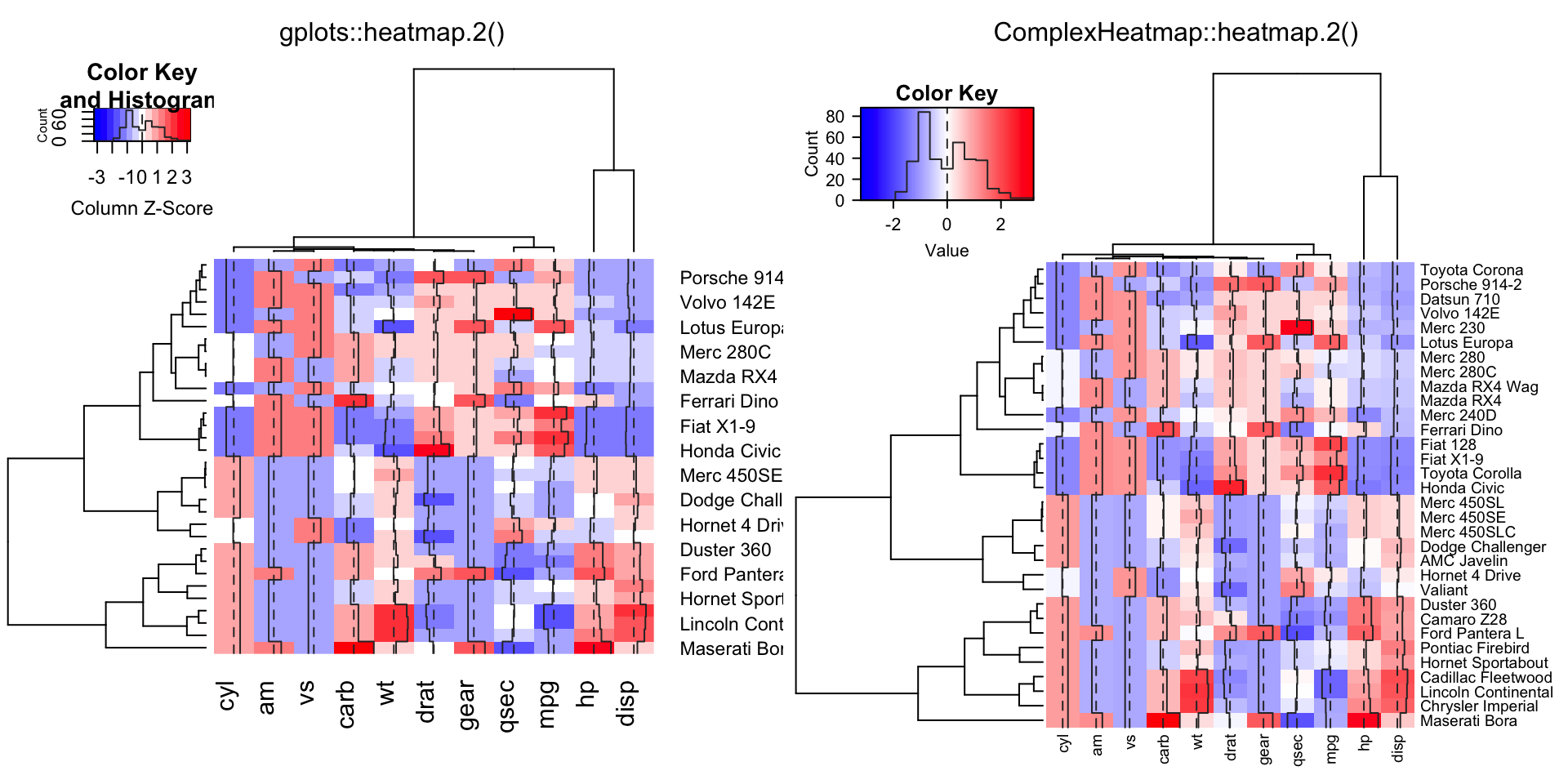compare_heatmap.2(x, col = cm.colors(255), scale = "column",
RowSideColors = rc, ColSideColors = cc, margin = c(5, 10),
xlab = "specification variables", ylab = "Car Models",
main = "heatmap(<Mtcars data>, ..., scale=\"column\")",
tracecol = "green", density = "density")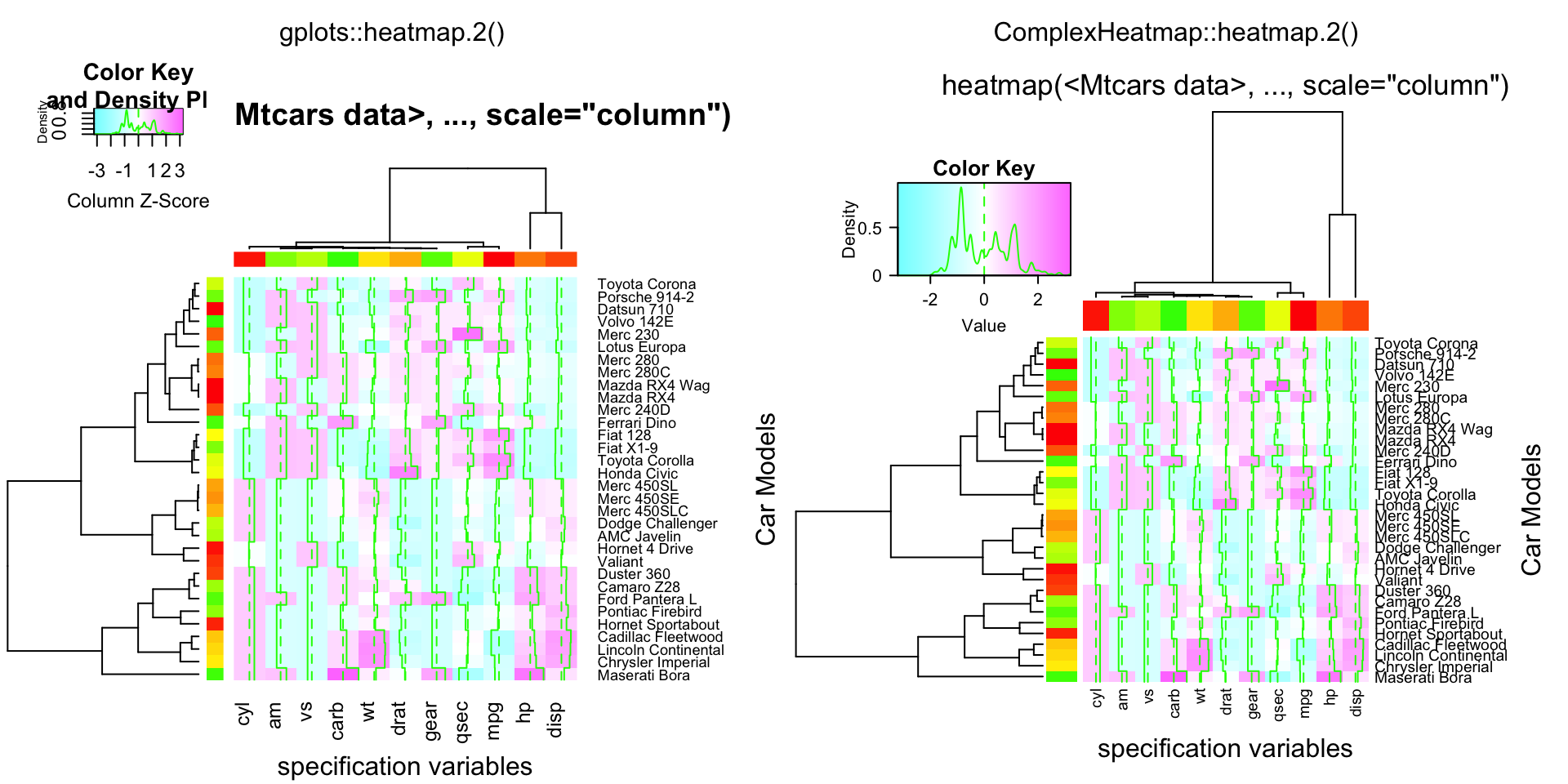compare_heatmap.2(x, col = cm.colors(255), scale = "column",
RowSideColors = rc, ColSideColors = cc, margin = c(5, 10),
xlab = "specification variables", ylab = "Car Models",
main = "heatmap(<Mtcars data>, ..., scale=\"column\")",
tracecol = "green", density = "density", colRow = rc, colCol = cc,
srtCol = 45, adjCol = c(0.5,1))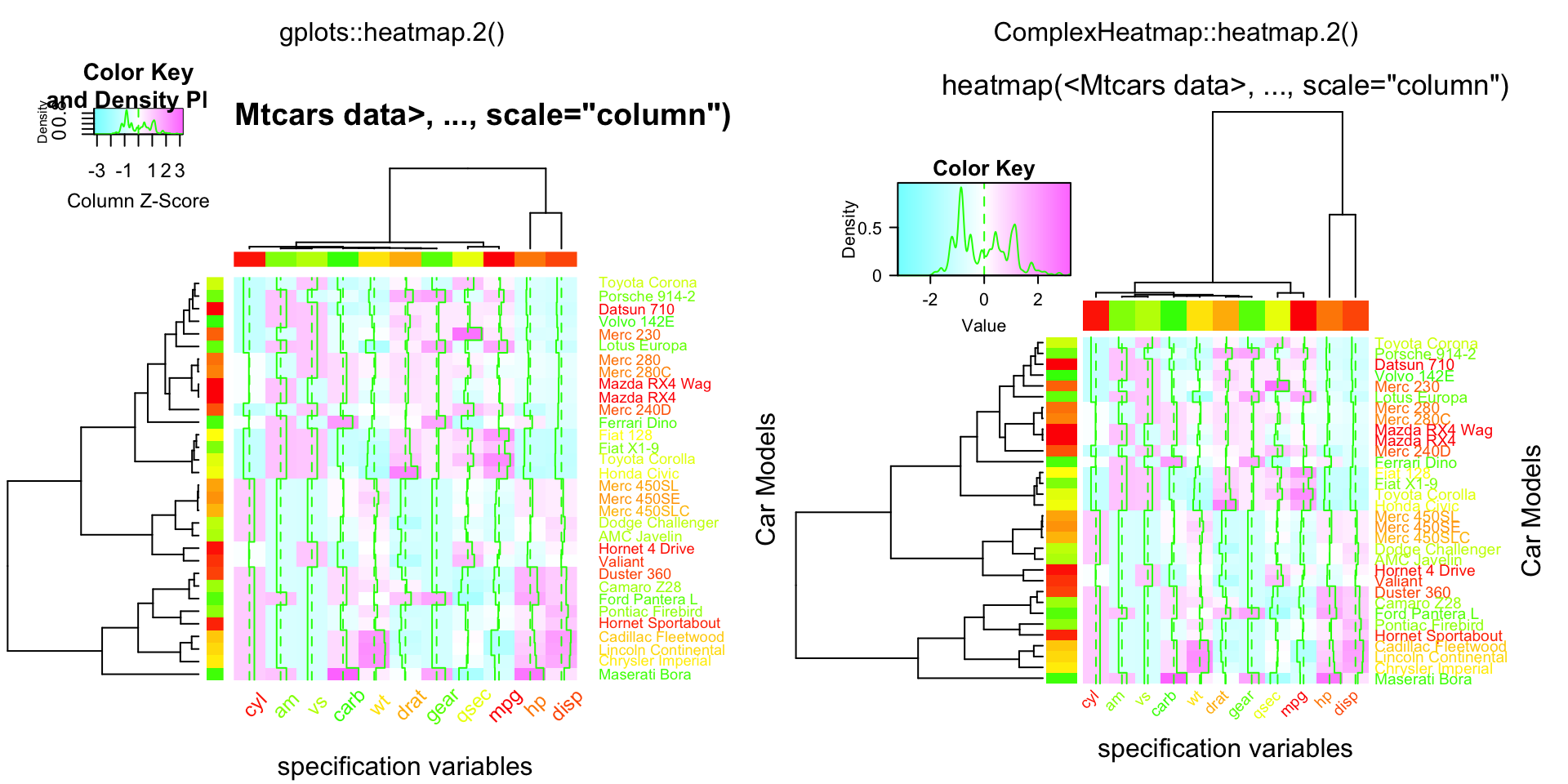data(attitude)
Ca = cor(attitude)

compare_heatmap.2(Ca, symm = TRUE, margin = c(6, 6), trace = "none" )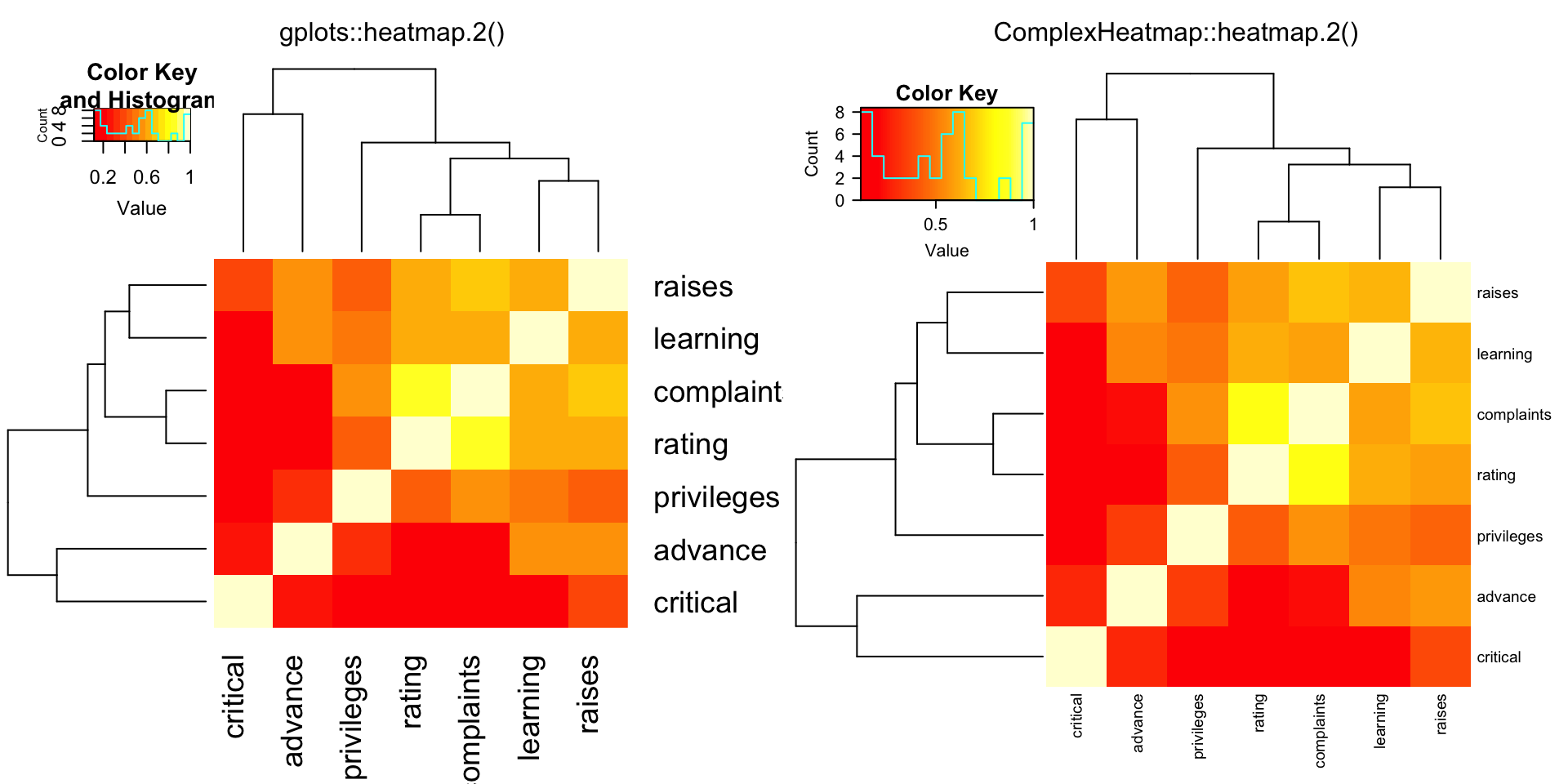compare_heatmap.2(Ca, Rowv = FALSE, symm = TRUE, margin = c(6, 6), trace = "none" )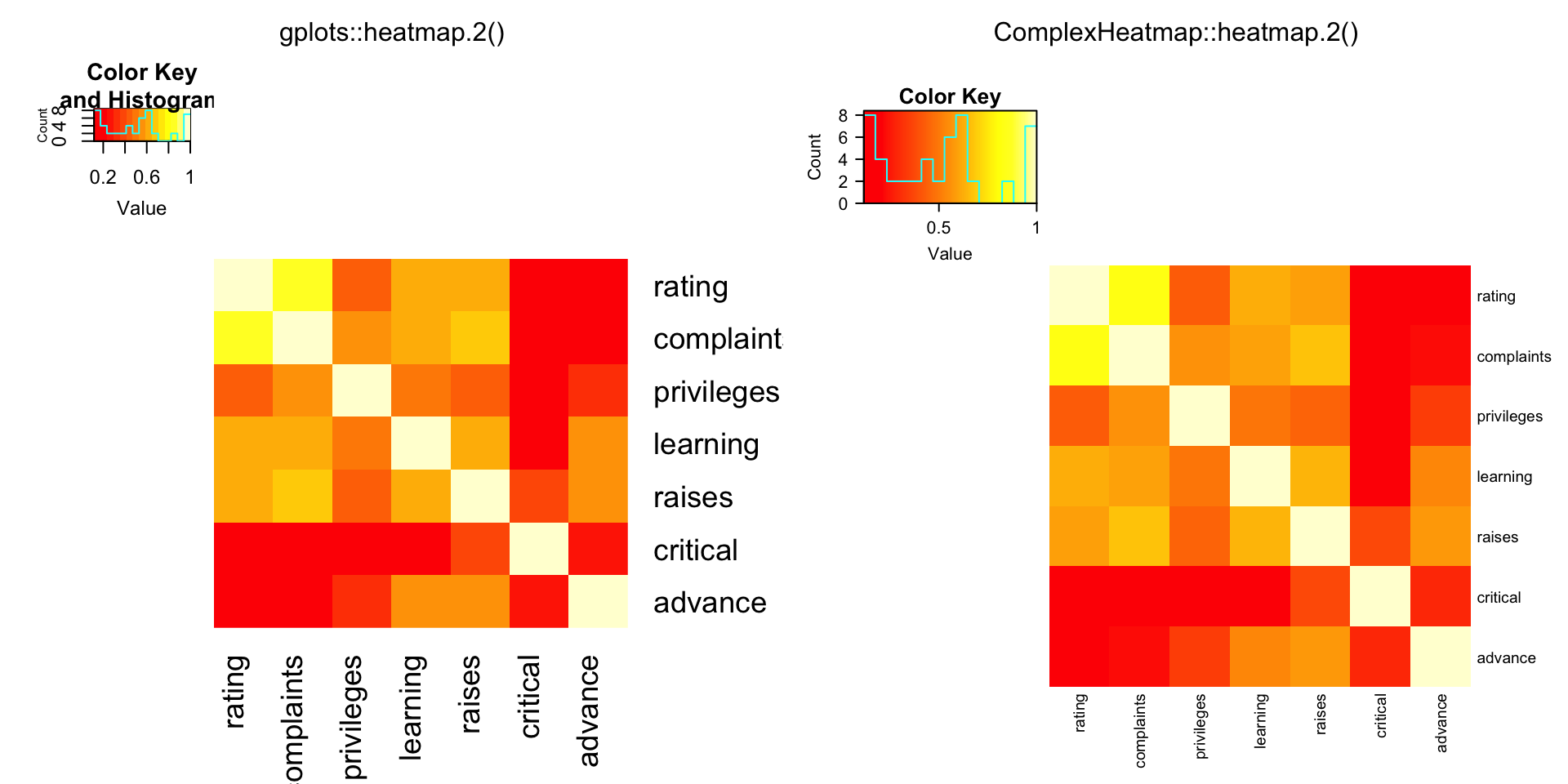data(USJudgeRatings)
cU = cor(USJudgeRatings)

compare_heatmap.2(cU, Rowv = FALSE, symm = TRUE, col = topo.colors(16),
distfun = function(c) as.dist(1 - c), trace = "none")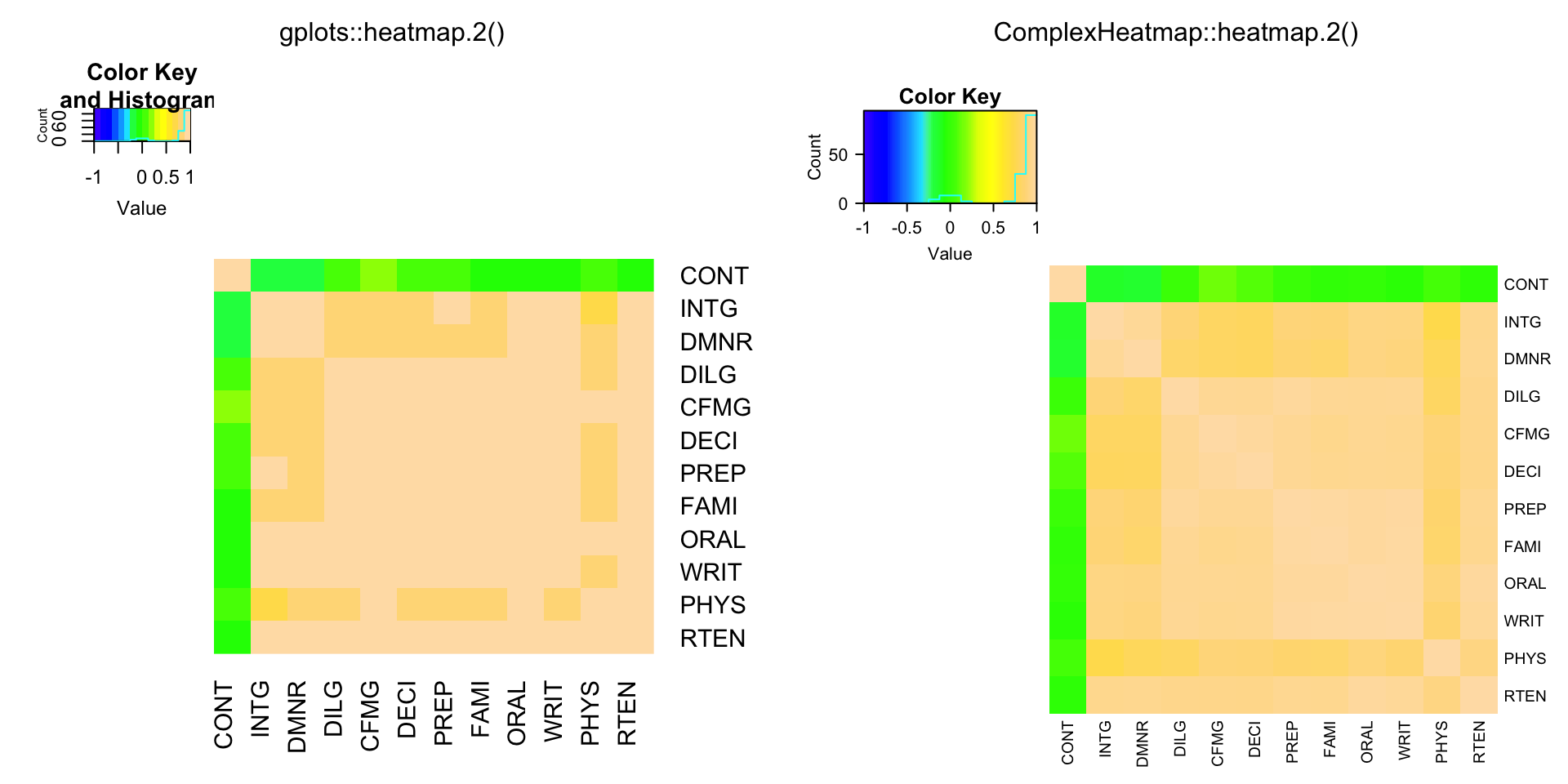hM = format(round(cU, 2))
compare_heatmap.2(cU, Rowv = FALSE, symm = TRUE, col = rev(heat.colors(16)),
distfun = function(c) as.dist(1 - c), trace = "none",
cellnote = hM)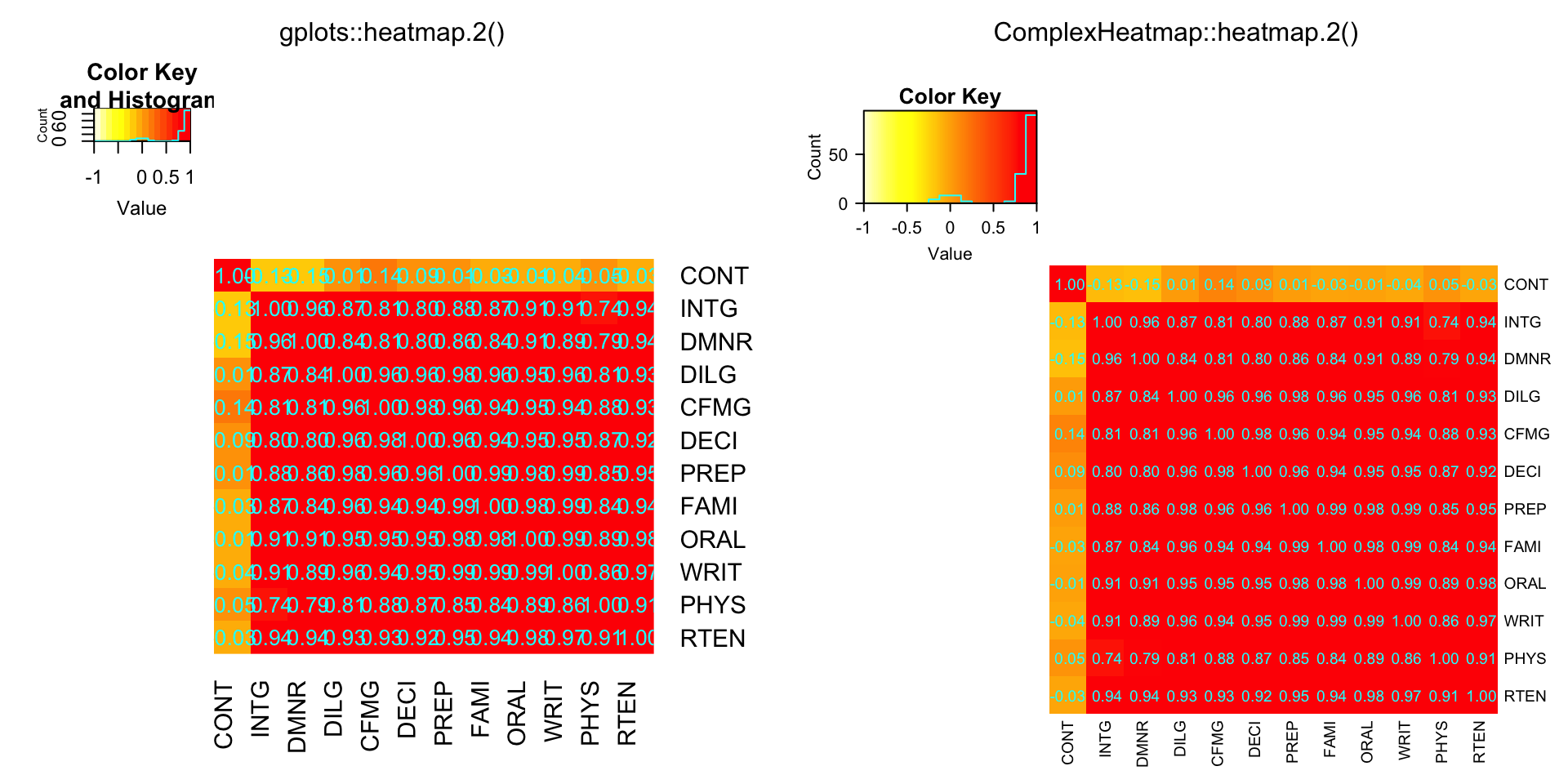library(affy)
data(SpikeIn)
pms = SpikeIn@pm

compare_heatmap.2(pms, col = rev(heat.colors(16)), main = "SpikeIn@pm",
xlab = "Relative Concentration", ylab = "Probeset",
scale = "row")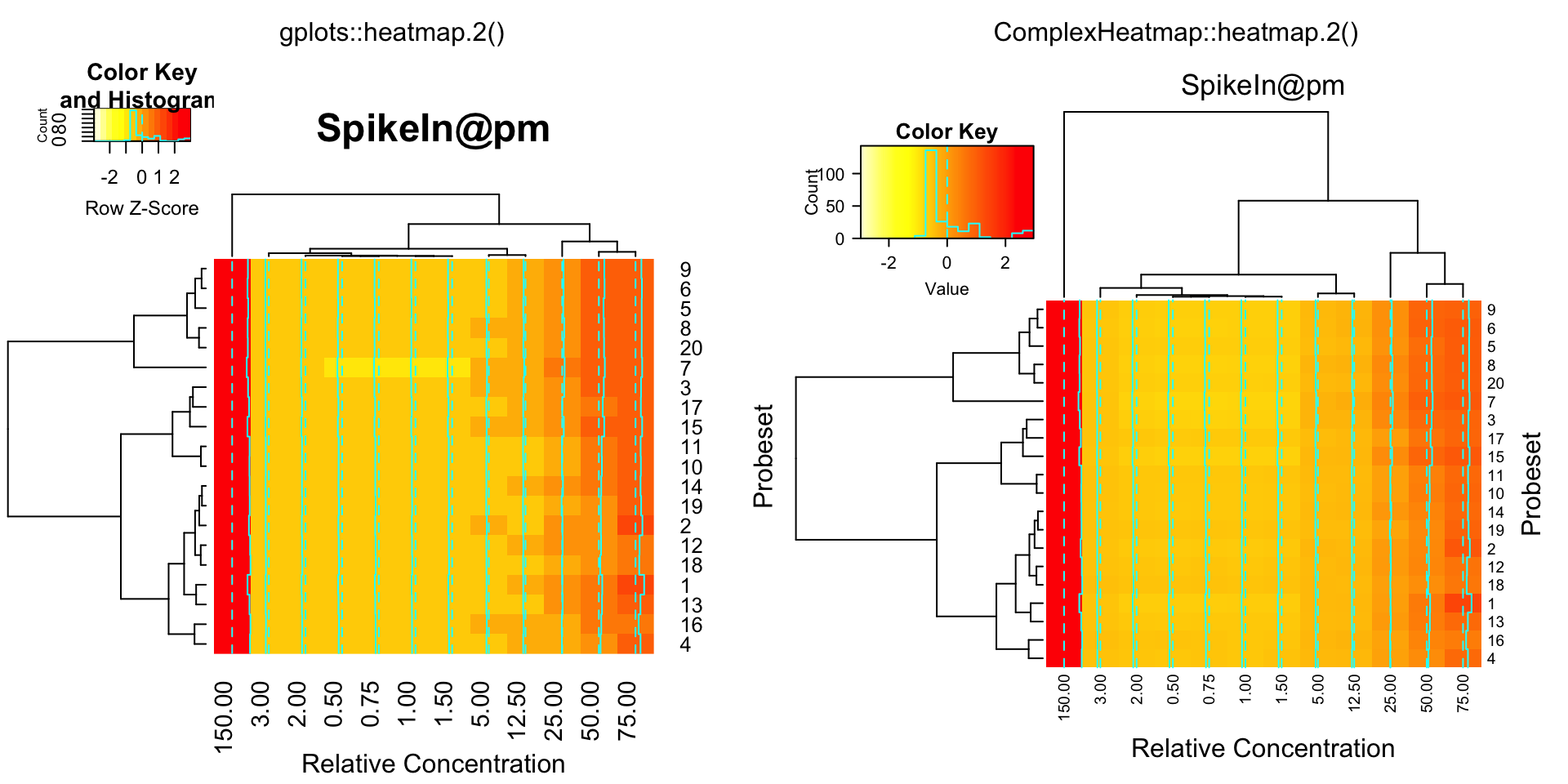data = pms / pms[, "12.50"]
data = ifelse(data > 1, data, -1 / data)
compare_heatmap.2(data, breaks = 16, col = gplots::redgreen, tracecol = "blue",
main = "SpikeIn@pm Fold Changes\nrelative to 12.50 sample",
xlab = "Relative Concentration", ylab = "Probeset")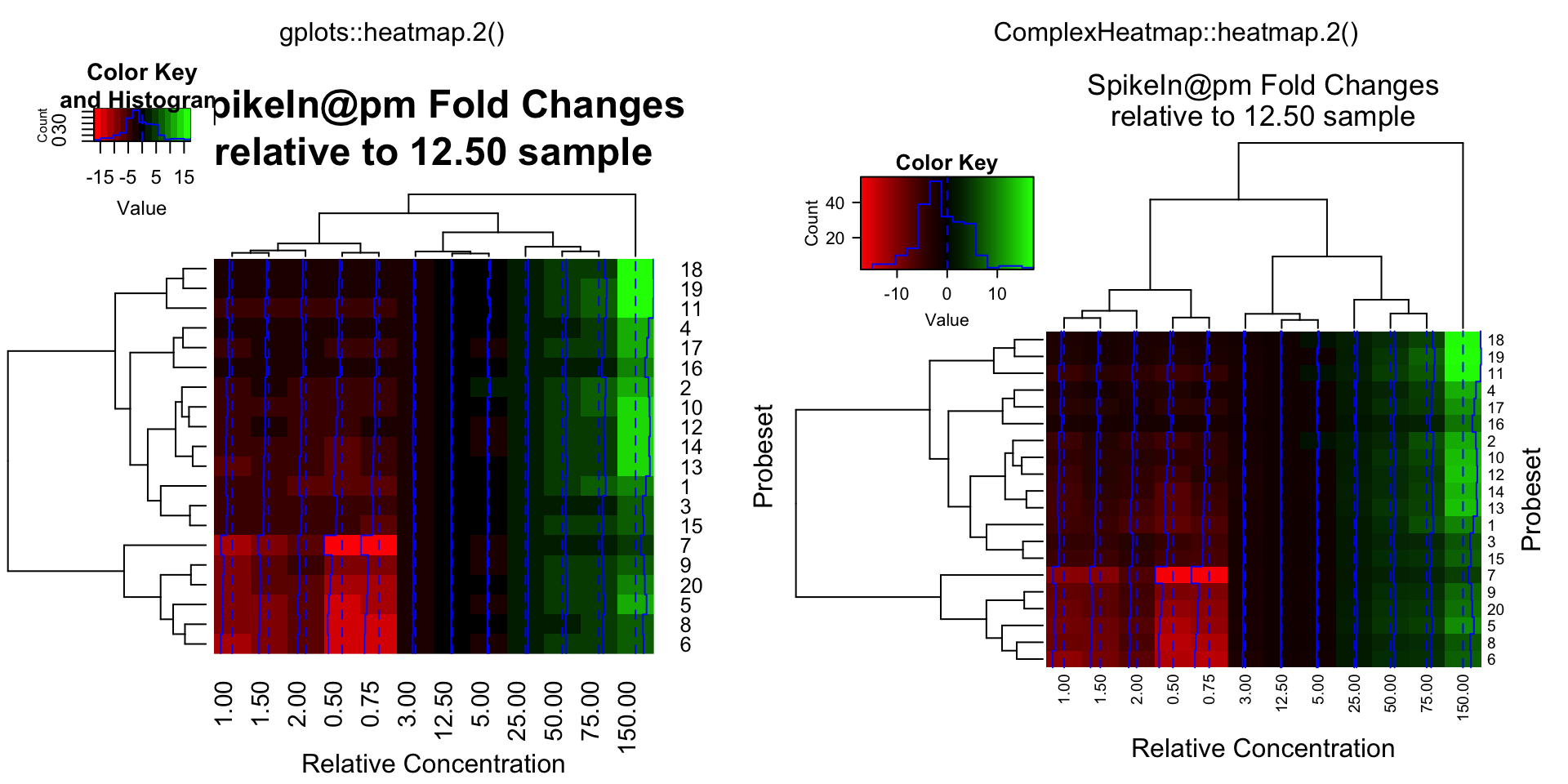## SessionInfo

sessionInfo()
## R version 4.0.2 (2020-06-22)
## Platform: x86_64-apple-darwin17.0 (64-bit)
## Running under: macOS Catalina 10.15.5
##
## Matrix products: default
## BLAS:   /Library/Frameworks/R.framework/Versions/4.0/Resources/lib/libRblas.dylib
## LAPACK: /Library/Frameworks/R.framework/Versions/4.0/Resources/lib/libRlapack.dylib
##
## locale:
##  en_GB.UTF-8/en_GB.UTF-8/en_GB.UTF-8/C/en_GB.UTF-8/en_GB.UTF-8
##
## attached base packages:
##  parallel  grid      stats     graphics  grDevices utils     datasets  methods   base
##
## other attached packages:
##  affy_1.66.0               Biobase_2.48.0            BiocGenerics_0.34.0
##  ComplexHeatmap_2.7.1.1012 knitr_1.30
##
## loaded via a namespace (and not attached):
##   Rcpp_1.0.5            BiocManager_1.30.10   compiler_4.0.2        RColorBrewer_1.1-2
##   zlibbioc_1.34.0       bitops_1.0-6          tools_4.0.2           digest_0.6.27
##   preprocessCore_1.50.0 evaluate_0.14         clue_0.3-57           png_0.1-7
##  rlang_0.4.8           magick_2.5.2          yaml_2.2.1            blogdown_0.17
##  xfun_0.19             stringr_1.4.0         cluster_2.1.0         GlobalOptions_0.1.2
##  S4Vectors_0.26.1      gridGraphics_0.5-0    gtools_3.8.2          caTools_1.18.0
##  IRanges_2.22.2        stats4_4.0.2          GetoptLong_1.0.4      rmarkdown_2.5
##  bookdown_0.21         magrittr_2.0.1        gplots_3.1.0          matrixStats_0.57.0
##  htmltools_0.5.0       shape_1.4.5           circlize_0.4.12.1004  colorspace_2.0-0
##  KernSmooth_2.23-18    stringi_1.5.3         crayon_1.3.4          rjson_0.2.20
##  affyio_1.58.0         Cairo_1.5-12.2